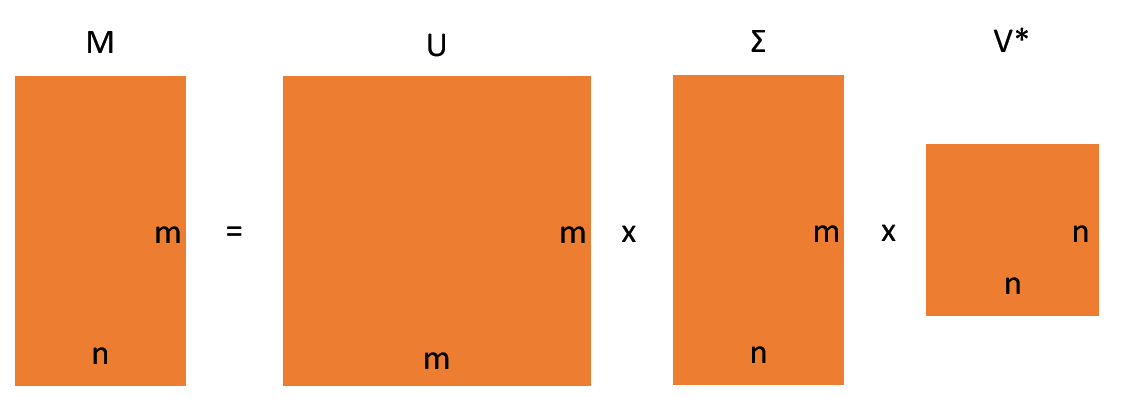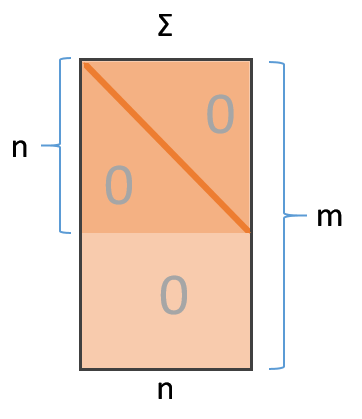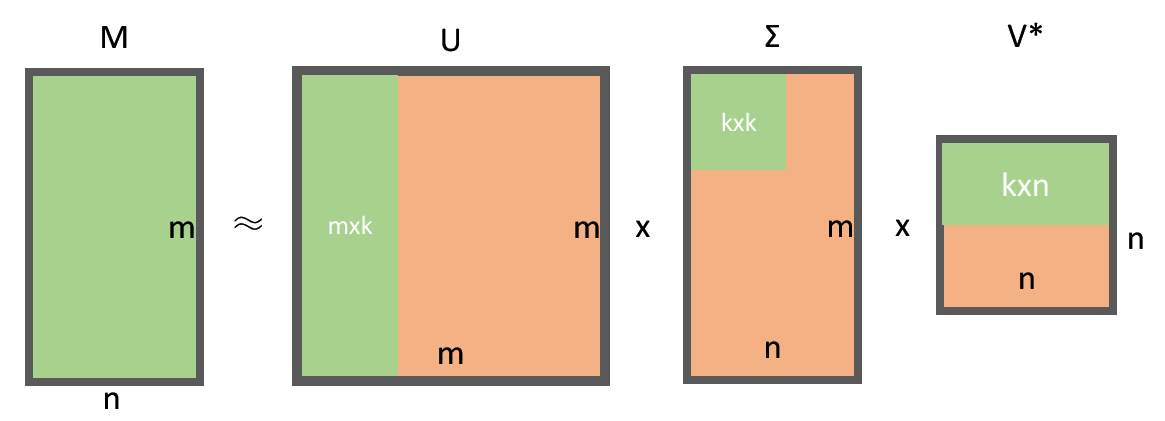PCA的大名如雷贯耳，曾经的我也以为PCA是个什么很复杂的东西，但是学习了线性代数之后才发现，PCA的原理简单而不失优雅，粗暴而不失趣味。

PCA是最易于理解的特征提取过程：通过对原始特征的线性组合构造新的“特征”，这些特征不同于原始特征，但是又能与原始特征一样表达原始数据的信息。

PCA (Primary Component Analysis, 主成分分析) 为什么叫做主成分分析呢？因为PCA构造出的新特征地位并不是等同的，即这些新特征的重要程度存在差异：

• 第一主成分 (the first component) 是新特征中最重要的特征，它在所有新特征中方差最大，这意味着它对数据变异的贡献是最大的；
• 第二主成分 (the second component) 在保证不影响第一主成分的基础上试图解释剩下的变异（即总变异 - 第一主成分引起的变异）；
• 第三主成分 (the third component) 在不保证第一和第二主成分呢的基础上试图解释剩下的变异（即总变异 - 第一主成分引起的变异 - 第二主成分引起的变异）;
• 依次类推……

# 线代原理

## 可对角化

n阶方阵A可对角化的充要条件是A每个特征值的几何重数与代数重数相等代数重数特征多项式中该特征值的幂次，几何重数指特征值对应的线性无关的特征向量的个数。

n阶方阵A可对角化的充要条件是A有n个线性无关的特征向量：几何重数与代数重数相等意味着n个线性无关的特征向量。

## 特征值分解

$$A=Q{\Lambda}Q^{-1}$$

• $Q$ 是n阶方阵，它的n个列向量是方阵A的n个特征向量
• $\Lambda$ 是对角方阵，对角线元素是方阵A的特征值，其位置与 $Q$ 中的特征向量位置相对应

## 奇异值分解

$$M=U{\Sigma}V^\ast$$

• U是 $m{\times}m$ 阶酉矩阵：U的m个列向量实际上是 $M^{\ast}M$ 的特征向量，称为M的左奇异向量
• $\Sigma$ 是 $m{\times}n$ 阶非负实数对角矩阵：对角线元素称为M的奇异值，一般情况下奇异值按从大到小的顺序排列！
• $V^\ast$ 是 $V$ 的共轭转置，是 $n{\times}n$ 阶酉矩阵：V的n个列向量实际上是 $MM^\ast$ 的特征向量，称为M的右奇异向量

$$A^\ast=(\overline{A})^T=\overline{A^T}$$

$$M=U{\Sigma}V^T$$

## 主成分分析

$$M_{m{\times}n}=U_{m{\times}m}{\Sigma_{m{\times}n}}V_{n{\times}n}^T$$$$M_{m{\times}n}=U_{m{\times}k}{\Sigma_{k{\times}k}}V_{k{\times}n}^T$$

# 工具

----- For reprint please indicate the source -----
0%